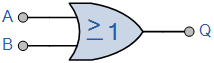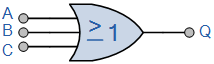A Logic OR Gate or Inclusive-OR gate is a type of digital logic gate that has an output which is normally at logic level “0” and only goes “HIGH” to a logic level “1” when one or more of its inputs are at logic level “1”.

The output, Q of a “Logic OR Gate” only returns “LOW” again when ALL of its inputs are at a logic level “0”. In other words for a logic OR gate, any “HIGH” input will give a “HIGH”, logic level “1” output.
The logic or Boolean expression given for a digital logic OR gate is that for Logical Addition which is denoted by a plus sign, ( + ) giving us the Boolean expression of:  A+B = Q.

Then we can define the operation of a 2-input logic OR gate as being:

“If either A or B is true, then Q is true”

2-input Transistor OR Gate

A simple 2-input logic OR gate can be constructed using RTL Resistor-transistor switches connected together as shown below with the inputs connected directly to the transistor bases. Either transistor must be saturated “ON” for an output at Q.Logic OR Gates are available using digital circuits to produce the desired logical function and is given a symbol whose shape represents the logical operation of the OR gate.

Digital Logic “OR” Gate Types

The 2-input Logic OR Gate

 Symbol Truth Table2-input OR Gate B A Q 0 0 0 0 1 1 1 0 1 1 1 1 Boolean Expression Q = A+B Read as A OR B gives Q

The 3-input Logic OR Gate

 Symbol Truth Table3-input OR Gate C B A Q 0 0 0 0 0 0 1 1 0 1 0 1 0 1 1 1 1 0 0 1 1 0 1 1 1 1 0 1 1 1 1 1 Boolean Expression Q = A+B+C Read as A OR B OR C gives Q

Like the AND gate, the OR function can have any number of individual inputs. However, commercial available OR gates are available in 2, 3, or 4 inputs types. Additional inputs will require gates to be cascaded together for example.

Multi-input OR GateThe Boolean Expression for this 6-input OR gate will therefore be:   Q = (A+B)+(C+D)+(E+F)
If the number of inputs required is an odd number of inputs any “unused” inputs can be held LOW by connecting them directly to ground using suitable “Pull-down” resistors.
Commonly available digital logic OR gate IC’s include:
TTL Logic OR Gates